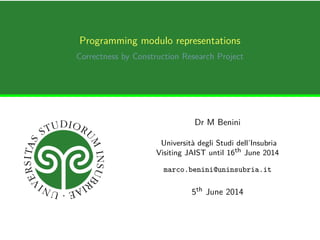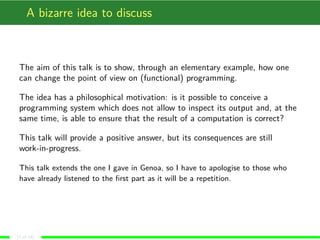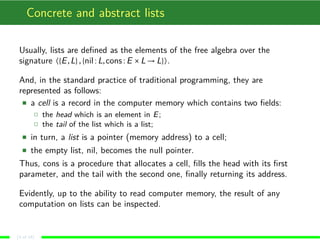Successfully reported this slideshow.

# Programming modulo representations

2

Share×
1 of 18
1 of 18

# Programming modulo representations

2

Share

This talk aims at introducing, through a very simple example, a way to represent data types in the λ-calculus, and thus, in functional programming languages, so that the structure of the data types itself becomes a parameter.

This very simple technical trick allows to reconsider programming as a way to express morphisms between models of a logical theory. As an application, it allows to realise a way to perform anonymous computations.

From a philosophical point of view, the presented approach shows how it is possible to conceive a real programming system where properties like correctness of programs can be proved, but data cannot be inspected, not even in principle.

This talk aims at introducing, through a very simple example, a way to represent data types in the λ-calculus, and thus, in functional programming languages, so that the structure of the data types itself becomes a parameter.

This very simple technical trick allows to reconsider programming as a way to express morphisms between models of a logical theory. As an application, it allows to realise a way to perform anonymous computations.

From a philosophical point of view, the presented approach shows how it is possible to conceive a real programming system where properties like correctness of programs can be proved, but data cannot be inspected, not even in principle.

## More Related Content

### Related Books

Free with a 14 day trial from Scribd

See all

### Related Audiobooks

Free with a 14 day trial from Scribd

See all

### Programming modulo representations

1. 1. Programming modulo representations Correctness by Construction Research Project Dr M Benini Università degli Studi dell’Insubria Visiting JAIST until 16th June 2014 marco.benini@uninsubria.it 5th June 2014
2. 2. A bizarre idea to discuss The aim of this talk is to show, through an elementary example, how one can change the point of view on (functional) programming. The idea has a philosophical motivation: is it possible to conceive a programming system which does not allow to inspect its output and, at the same time, is able to ensure that the result of a computation is correct? This talk will provide a positive answer, but its consequences are still work-in-progress. This talk extends the one I gave in Genoa, so I have to apologise to those who have already listened to the ﬁrst part as it will be a repetition. (2 of 18)
3. 3. Concrete and abstract lists Usually, lists are deﬁned as the elements of the free algebra over the signature 〈{E,L},{nil:L,cons:E ×L → L}〉. And, in the standard practice of traditional programming, they are represented as follows: a cell is a record in the computer memory which contains two ﬁelds: the head which is an element in E; the tail of the list which is a list; in turn, a list is a pointer (memory address) to a cell; the empty list, nil, becomes the null pointer. Thus, cons is a procedure that allocates a cell, ﬁlls the head with its ﬁrst parameter, and the tail with the second one, ﬁnally returning its address. Evidently, up to the ability to read computer memory, the result of any computation on lists can be inspected. (3 of 18)
4. 4. Concrete manipulation of a list As an example of program, we consider the concatenate function. Its speciﬁcation is: “Given the lists [x1,...,xn] and [y1,...,ym], concatenate has to return the list [x1,...,xn,y1,...,ym]”. Usually, it is implemented as concatenate(x,y) ≡ if x = nil then return y else q := x while q = nil do p := q q := q → tail p → tail := y return x (4 of 18)
5. 5. Correctness The previous algorithm is correct. In fact, when x = nil, it returns y, satisfying the speciﬁcation. When x = nil, x = [x1,...,xn]. So, at the end of the i-th iteration step, p = [xi ,...,xn] and q = [xi+1,...,xn], as it is immediate to prove by induction. Also, the cycle terminates after n iterations, and p = [xn]. But, in the concrete representation of x, p → tail must be nil and the assignment p → tail := y substitutes nil with y. So x becomes [x1,...,xn,y1,...,ym], as required. The proof sketched above uses in an essential way the concrete representation of the x list, because the algorithm uses “list surgery”. The algorithm, as one deduces from the proof, computes in O (|x|) steps, and uses a constant amount of memory, apart the one used the represent the input. (5 of 18)
6. 6. A functional derivation Dropping list surgery, we can use the abstract formalisation of lists directly: concatenate(x,y) ≡ if x = nil then return y else return cons (hdx) (concatenate(tlx,y)) where hd and tl return the head and the tail of its argument, respectively. Of course, this is a functional program, and it is justiﬁed by the following reasoning, which can be immediately converted into a formal correctness proof by induction on the structure of x: 1. we want that concatenate([x1,...,xn],[y1,...,ym]) = [x1,...,xn,y1,...,ym], 2. as before, if x = nil, the result is just y 3. when x = nil, concatenate([x1,...,xn],[y1,...,ym]) yields the same result as consx1 (concatenate([x2,...,xn],[y1,...,ym])); 4. in the line above, the recursive application decreases the length of the ﬁrst argument, so recursion terminates after n steps, yielding the result. (6 of 18)
7. 7. Recursion versus induction In the functional implementation of concatenate, we may interpret the recursive schema as the computational counterpart of an inductive schema. It is immediate to see that such an inductive schema becomes the skeleton of the correctness proof. So, the functional program “carries” with itself its proof of correctness, in some sense. Usually, the functional implementation of concatenate is regarded as ineﬃcient because it recursively constructs a number of intermediate lists before yielding the ﬁnal results. That is, the functional program computes in O (|x|) steps, but it uses O |x|2 memory cells in a plain implementation of the language. To inspect the result we need to know that nil and cons are the constructors of the data type of lists, a piece of knowledge that is shared between the user and the programmer. (7 of 18)
8. 8. Abstracting over lists We formalised a list [x1,...,xm] as consx1 (consx2 (...(consxm nil)...)). We can use a slightly diﬀerent representation1: λn,c. c x1 (c x2 (...(c xm n)...)) . The key idea is to abstract over the structure of the data type, making it part of the representation of the datum. Alternatively, we can interpret this representation A as the abstract datum, and the concrete one, C can be obtained by passing the instances of the constructors to A. For example, the standard formalisation is obtained by (Anilcons). 1As far as I know, the general algorithm to derive such a representation is due to Böhm and Berarducci, and it can be traced back to Church. But the paper of Böhm and Berarducci is subtle as it relies on a typed λ-system. (8 of 18)
9. 9. Abstracting one step further In fact, it is possible, by assuming that the λ-calculus (type theory) we are using has pairs, to abstract a bit further, so to completely hide the data type. Instead of writing [x1,...,xm] as λn,c. c x1 (c x2 (...(c xm n)...)) , we may substitute the constructors with the data type a, which is a 2-tuple, the ﬁrst element being the concrete representation for nil, the second being the concrete representation for cons: λa. π2 ax1 (π2 ax2 (...(π2 axm (π1 a))...)) , where π1 and π2 are the standard projections. In this way, the programmer does not know how the list is concretely represented, but simply that the ﬁrst element of a is how to interpret nil and the second element of a is how to represent cons. (9 of 18)
10. 10. Interpreting abstract lists An abstract list can be thought of as representing a term in the ﬁrst-order logical language with the equality relation symbol, and the signature of the data type of lists. The λ-term standing for the abstract list realises the mapping from the logical term — the list, the body of the abstraction — into some model, which is speciﬁed when we apply to the λ-term the way to interpret the function symbols, which, in turn, are not speciﬁed. If we ﬁx this point of view, we can write a “correct by construction” implementation of concatenate: concatenate ≡ λx,y,a. x (y a),(π2 a) . (10 of 18)
11. 11. Correctness by construction I concatenate ≡ λx,y,a. x (y a),(π2 a) It is worth explaining the construction of this program: 1. it is a function, which takes two argument x and y; 2. it returns an abstract list, so a λ-term of the form λa. L, with L a logical term in the language of lists, the constructors represented as projections from the signature a; 3. the y abstract list gets interpreted in the same model as the result of concatenate — and this is rendered by (y a); 4. the x abstract list gets interpreted in a model which has the same interpretation for cons, (π2 a), but it interprets nil as the ‘concrete’ y. We should remark that, in fact, this abstract implementation is, in essence, the very same algorithm we have shown in the beginning, deprived from the irrelevant details about the concrete data structure of lists. So, it is an eﬃcient functional implementation. (11 of 18)
12. 12. Correctness by construction II concatenate ≡ λx,y,a. x (y a),(π2 a) The above deﬁnition is a direct coding of the explanation. In turn, the explanation can be converted into a correctness proof by observing that the structure depicted in point (4) is a model for the theory of lists; there is a mapping that preserves the meaning between the standard term model and the model above; this mapping is just the function concatenate. The idea behind this proof is that the function concatenate, intended as a program, is nothing but a morphism between models of the same theory. A non-evident aspect of the explanation of concatenate is that it correctly operates in any model for the theory of lists. (12 of 18)
13. 13. One program, many meanings For example, natural numbers, described as the structure generated by zero and successor, are a model for lists: cons ≡ λe,l. sucl and nil ≡ 0. And concatenate becomes just the usual addition. For example, interpreting cons as the Cartesian product and nil as the terminal object in a category with products, we get another model for lists. And concatenate becomes just the Cartesian product of two products. For example, interpreting cons as function application and nil as the identity function, we get another model for lists. And concatenate becomes function composition. And, in all these cases, the programmer is not aware of what his program is actually computing. But, still, as far as he assumes that there is morphism between the standard representation of lists and the intended concrete structure the program will operate on, he will be able to prove that his program is correct. (13 of 18)
14. 14. Interpretations and computing Suppose to have three actors: the real user of the program; the programmer; and a malicious user of the program. Since the real user can invoke the program by providing the inputs x and y, but not the concrete interpretation, he will obtain an abstract result which is a program that takes as input just the concrete representation a, something he can use locally and privately. The programmer knows that the purpose of the program is to concatenate lists, and he is able to write a correct implementation, even if he does not know how lists are concretely represented. So, he cannot inspect the output of the user, but he is able to test the program in the usual way by employing a standard representation for lists. The malicious user, who wants to steal the result of the real user, can inspect x and y, as well as the program, but he does not know a, as the real user does not provide it. So he can inspect the abstract result, but he will be unable to understand its meaning in the world of the real user. (14 of 18)
15. 15. Generalising Does it work only for lists? The theory behind the abstract representation for data types has been developed by Böhm and Berarducci, and it directly applies to all the data structures that can be formalised as free algebras of terms over a ﬁrst-order signature. This holds for a large number of the elementary structures which are used in the current practice of programming. In a similar way, co-inductive data structures can be modelled as well. For data structures which are not free (co-)algebras, there are still some open problems, but, to some extent, they can be modelled in the same spirit — essentially, most data types used in programming are quotients of free (co-)algebras, so the inductive pattern still works, that is, recursion on the structure of the free (co-)algebra is a correct way to perform computation, even if not necessarily eﬃcient. Does it work in a “real” programming language? As far as the programming language supports the dynamic creation of functions, e.g., by providing abstraction, the technique can be immediately used. This is the case for any functional language. (15 of 18)
16. 16. A philosophical remark Any program which takes as input the description of the data types it uses, in the abstract sense we introduced earlier, automatically computes modulo a concrete representation. Nothing prevents to use arbitrary representations: as far as one can provide a morphism from the free (co-)algebra of terms to the intended model, the result will be correctly computed. Using a bizarre representation hides the result to the programmer and to any other user who does not know the morphism that maps the abstract result into its concrete representation. So, this technique, in principle, may provide a way to perform anonymous correct computations. On another side, nothing prevents from using a non-computable concrete representation: in this way, the result cannot be inspected even by the user, although he perfectly knows, by means of a mathematical proof, that it is correct. So, inspectability and computability are distinct concepts and, in particular, the latter does not imply the former. (16 of 18)
17. 17. Conclusions In the previous slide there is a hidden assumption: that the logical theory has a canonical model which can be transformed into a any other model via a suitable mapping. This is not true in general. So the presented point of view can be stretched only when considering logical theories having such a classifying model — which is the case for free (co-)algebras of terms, for example. In my recent research (and my previous talk here), I’ve shown a semantics for ﬁrst-order intuitionistic logical theories, based on a categorical setting, which has classifying models. So, every such a theory could, in principle, be regarded as a “data type” in the sense of this talk. Of course, much work has to be done... so any hint, suggestion, critique, question is mostly welcome! (17 of 18)
18. 18. The end Harmony — © Marco Benini (2014) (18 of 18)